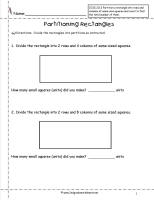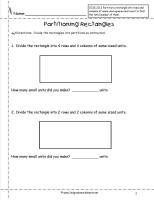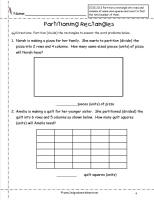﻿ Partition Rectangles Worksheets, CCSS 2.G.2

# Common Core State Standard 2.G.2

## Geometry

### Reason with shapes and attributes.

2. Partition a rectangle into rows and columns of same-size squares and count to find the total number of them.

###Partition Rectangles Worksheet

Common Core State Standards: 2.G.2
Geometry
Reason with shapes and attributes.

Partition a rectangle into rows and columns of same-size squares and count to find the total number of them.

###Partition Rectangles Worksheet

Common Core State Standards: 2.G.2
Geometry
Reason with shapes and attributes.

Partition a rectangle into rows and columns of same-size squares and count to find the total number of them.

###Partition Rectangles Worksheet

Common Core State Standards: 2.G.2
Geometry
Reason with shapes and attributes.

Partition a rectangle into rows and columns of same-size squares and count to find the total number of them.

###Partition Rectangles Worksheet

Common Core State Standards: 2.G.2
Geometry
Reason with shapes and attributes.

Partition a rectangle into rows and columns of same-size squares and count to find the total number of them.